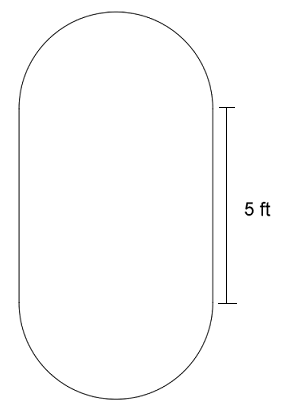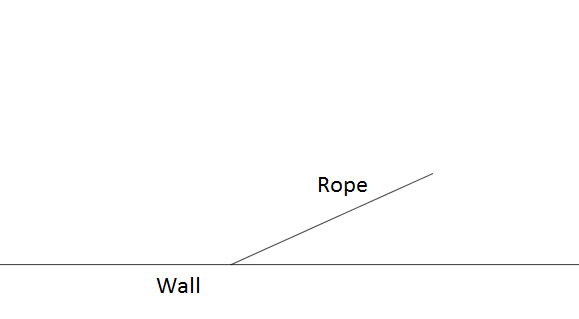Match Fishtank is now Fishtank Learning!

# Geometry

## Objective

Solve real-world and mathematical problems using the relationship between the area of a circle and its radius.

## Common Core Standards

### Core Standards

?

• 7.G.B.4 — Know the formulas for the area and circumference of a circle and use them to solve problems; give an informal derivation of the relationship between the circumference and area of a circle.

## Criteria for Success

?

1. Know the formula that relates the area and radius of a circle: ${{A=\pi r^2}}$.
2. Use the formula ${{A=\pi r^2}}$ to solve problems.

## Tips for Teachers

?

Lessons 8 and 9 focus on the relationship between a circle’s area and its radius. In Lesson 9, students will solve real-world and mathematical problems involving area. Students will solve more problems involving all measurements of circles in Lessons 10 and 11.

#### Remote Learning Guidance

If you need to adapt or shorten this lesson for remote learning, we suggest prioritizing Anchor Problem 1 (can be done independently). Find more guidance on adapting our math curriculum for remote learning here.

#### Fishtank Plus

• Problem Set
• Student Handout Editor
• Vocabulary Package

## Anchor Problems

?

### Problem 1

Suzanne is making a circular table of out a square piece of wood. The diameter of the circle she is cutting is 6 feet. How much waste will she have for this project? Express your answer to the nearest square foot.

#### References

EngageNY Mathematics Grade 7 Mathematics > Module 3 > Topic C > Lesson 17Example 3

Grade 7 Mathematics > Module 3 > Topic C > Lesson 17 of the New York State Common Core Mathematics Curriculum from EngageNY and Great Minds. © 2015 Great Minds. Licensed by EngageNY of the New York State Education Department under the CC BY-NC-SA 3.0 US license. Accessed Dec. 2, 2016, 5:15 p.m..

Modified by Fishtank Learning, Inc.

### Problem 2

Recall the image below from Lesson 7, composed of a square and two semi-circles. Find the area of the figure.### Problem 3

A 12 ft. rope is attached to the wall of a garage. A dog’s collar is attached to the end of the rope to allow him to safely stay outside. What is the area of the space the dog has outside?## Problem Set

?The following resources include problems and activities aligned to the objective of the lesson that can be used to create your own problem set.

• Include problems with composite shapes including simple quadrilaterals, triangle, and circles or semi-circles.
• Include problems where students determine the percent increase in area from one circle to another; for example, a medium pizza has a diameter of 14 inches and a large pizza has a diameter of 16 inches. By what percent is the area of the large pizza greater than the medium pizza?